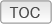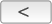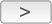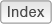Cakewalk // Documentation // SONAR X2 // ComparisonComparison

The following table highlights the key differences between SONAR X2 Producer, SONAR X2 Studio, and SONAR X2 Essential.
 Product Comparison SONAR X2 Essential SONAR X2 Studio SONAR X2 Producer General Max number of audio tracks 64 Unlimited Unlimited Max number of MIDI tracks Unlimited Unlimited Unlimited Max Bit Depth / Sample Rate 64-bit float / 384kHz 64-bit float / 384kHz 64-bit float / 384kHz Max number of effects / instruments 64 / 32 Unlimited Unlimited Max simultaneous buses 16 Unlimited Unlimited Max audio effects sends (per track/bus) 5 Unlimited Unlimited Internal mixing engine 64-bit 64-bit 64-bit Windows x64 Support Native x64 Native x64 Native x64 Step Sequencer 2.0 x x x Video import/export x x x GPU Acceleration x x x Matrix view x x x Arpeggiator x x x FX Chains x x x FX Chain UI customizing x x External Insert x x AudioSnap 2.0 x x V-Vocal 1.5 x x Surround Sound x x SoundCloud export x x x MusicXML export x x x Automation record modes x x R-MIX SONAR x ProChannel x Audio plug-in effects Alias Factor x x x Analyst x x x Bit Meter x x x Boost 11 Peak Limiter x x x Classic Phaser x x x Compressor/Gate x x x CW - Delay (Mono) x x x CW- EQ (Mono) x x x CW- Reverb (Mono) x x x DSP-FX Chorus (32-bit Only) x x x DSP-FX Delay (32-bit Only) x x x DSP-FX EQ (32-bit Only) x x x DSP-FX Flange (32-bit Only) x x x DSP-FX StudioVerb (32-bit Only) x x x FX1 Dynamics Processor x x x FX2 AmpSim x x x FX2 TapeSim x x x HF Exciter x x x Modfilter x x x Multivoice Chorus/Flanger x x x Parametric EQ x x x Para-Q x x x Pitch x x x Reverb x x x SpectraFX x x x Stereo Delay (32-bit Only) x x x Stereo Reverb x x x Tempo Delay x x x Sonitus fx: EQ x x x Sonitus fx: Reverb x x x Sonitus fx: Compressor x x Sonitus fx: Delay x x Sonitus fx: Gate x x Sonitus fx: Modulator x x Sonitus fx: Multiband x x Sonitus fx: Surround x x Sonitus fx: Surround Compressor x x Sonitus fx: Wah Wah x x Sonitus fx:Phase x x Channel Tools x x TH2 SONAR x x TH2 Producer x BREVERB SONAR x LP-64 EQ x LP-64 Multiband x Perfect Space (32-bit Only) x PX-64 Percussion Strip x TL-64 Tube Leveler x TS-64 Transient Shaper x Vintage Channel x VX-64 Vocal Strip x MIDI plug-in effects MFX Arpeggiator x x x MFX Change Velocity x x x MFX Chord Analyzer x x x MFX Echo/Delay x x x MFX Event Filter x x x MFX Quantize x x x MFX Transpose x x x Instruments Cakewalk Sound Center x x x Cakewalk TTS-1 x x x Dimension LE LE Pro Dreamstation DXi (32-bit Only) x x x DropZone x x x Square I x x x Studio Instruments Suite x x x Cyclone x x Pentagon I x x PSYN II x x Rapture x Rapture LE x Roland Groove Synth x x x RXP REX Player x x SFZ Sound Font Player x x Z3ta+ x x Session Drummer 3 x True Pianos Amber (x86, x64) x Expansion Packs for Dimension Pro Digital Sound Factory V2 Classic Keys x Hollywood Edge FX x Dimension Pro Expansion Pack 1 x Dimension Pro Expansion Pack 2 x Classic Sounds for Dimension Pro x Audio Loops, MIDI Clips & Projects 500 MB REX Loopmasters x 500 MB REX/WAV Sample Magic x 210 MB WAV XMIX x MIDI Groove Clips x Tutorial Projects x Artist demo projects x